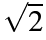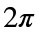# Problem 55230. Find Closest Constant

Given a number x, return the value that is closest to x from this list of constants: 0, 1,, e, π,(also known as τ).
For example:
c = closestconst(1.5)
c =
1.4142
c = closestconst(2.5)
c =
2.7183
c = closestconst(50)
c =
6.2832
c = closestconst(-10)
c =
0
If x is equally close to two values (eg), you can return either value (so either 1 orwould be correct in this example).

### Solution Stats

48.17% Correct | 51.83% Incorrect
Last Solution submitted on Dec 01, 2023

### Community Treasure Hunt

Find the treasures in MATLAB Central and discover how the community can help you!

Start Hunting!